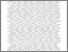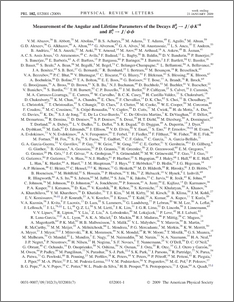# Measurement of the angular and lifetime parameters of the decays $B_{d}^{0}\to J/ψK^{*0}$ and $B_{s}^{0}\to J/ψφ$

Collaboration, D0 and Bertram, Iain and Borissov, Guennadi and Fox, Harald and Williams, Mark and Ratoff, Peter and Love, Peter and Rakitin, Alexander and Sopczak, Andre (2009) Measurement of the angular and lifetime parameters of the decays $B_{d}^{0}\to J/ψK^{*0}$ and $B_{s}^{0}\to J/ψφ$. Physical review letters, 102 (3). ISSN 1079-7114Preview
PDF
PhysRevLett.102.032001.pdf - Published Version

## Abstract

We present measurements of the linear polarization amplitudes and the strong relative phases that describe the flavor-untagged decays $B_{d}^{0}\to J/\psi K^{*0}$ and $B_{s}^{0}\to J/\psi\phi$ in the transversity basis. We also measure the mean lifetime $\bar{\tau}_{s}$ of the $B_{s}^{0}$ mass eigenstates and the lifetime ratio $\bar{\tau}_{s}/\tau_{d}$. The analyses are based on approximately 2.8 fb$^{-1}$ of data recorded with the D0 detector. From our measurements of the angular parameters we conclude that there is no evidence for a deviation from flavor SU(3) symmetry for these decays and that the factorization assumption is not valid for the $B_{d}^{0}\to J/\psi K^{*0}$ decay.

Item Type:
Journal Article
Journal or Publication Title:
Physical review letters
© 2009 The American Physical Society 7 pages, 4 figures. Submitted to Phys. Rev. Lett
Uncontrolled Keywords:
/dk/atira/pure/subjectarea/asjc/3100
Subjects:
Departments:
ID Code:
63762
Deposited By:
Deposited On:
30 Apr 2013 10:53
Refereed?:
Yes
Published?:
Published